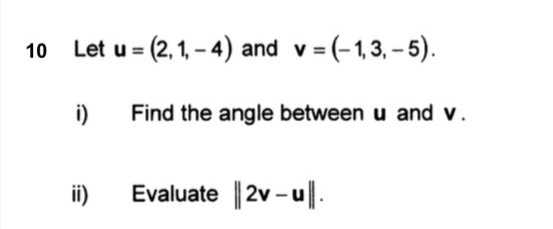### ¿Todavía tienes preguntas de matemáticas?

Pregunte a nuestros tutores expertos
Algebra
Pregunta$$10$$ Let $$u = ( 2,1 , - 4 )$$ and $$v = ( - 1,3 , - 5 )$$ i) Find the angle between $$u$$ and $$v$$ ii) Evaluate $$\| 2 v - u \|$$

i):39.23$$°$$
ii):$$\sqrt{77}$$